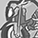# New to Qlik Sense

If you’re new to Qlik Sense, start with this Discussion Board and get up-to-speed quickly.

Announcements
Our May 2021 end-to-end product release from Data Integration to Data Analytics is out! READ DETAILS
cancel
Showing results for
Did you mean:Contributor III

## Set analysis on dimension in aggr() funtion

Hi Everyone,

I am stuck with aggr() function issue in QlikSense App. I am using Aggr() function in my expression, and inside the measure, I have restricted few filters while calculating it using set analysis, the same filter restriction should apply to the dimension as well. I tried using Set analysis on dimension, but it doesnot work . Please help .

Below is my expression :

aggr(if(RangeSum(Above(SUM({<Period=,Date={">=01/01/2019<=30/06/2019"}>}SPEND_ACTUAL), 0, RowNo()))/sum( {<Period=,Date={">=01/01/2019<=30/06/2019"}>} TOTAL SPEND_ACTUAL)<=0.80,PARENT_SUPPLIER_ID),
(PARENT_SUPPLIER_ID,(=SUM({<Period=,Date={">=01/01/2019<=30/06/2019"}>}SPEND_ACTUAL),Desc)))

In this expression, I want to avoid filtering PArent_Supplier_ID diemsion on Period as well but it is not working , Because of that even when I applied restriction on whole measure, the measure still filters on PEriod and calculates the wrong value.

2 RepliesMVP

If you are not using calculated dimension, i.e. - using a field directly in dimension in a chart, the restriction using set analysis in the main measure expression should limit the dimension values as well. What are your dimension and measure expression?Contributor III
Author

My dimension = (PARENT_SUPPLIER_ID,(=SUM({<Period=,Date={">=01/01/2019<=30/06/2019"}>}SPEND_ACTUAL),Desc)))

and measure  = if(RangeSum(Above(SUM({<Period=,Date={">=01/01/2019<=30/06/2019"}>}SPEND_ACTUAL), 0, RowNo()))/sum( {<Period=,Date={">=01/01/2019<=30/06/2019"}>} TOTAL SPEND_ACTUAL)<=0.80,PARENT_SUPPLIER_ID)

Set analysis "{<Period=,Date={">=01/01/2019<=30/06/2019"}" being applied in dimension while doing sum , but while calculating the aggregated value, it  is restricting PArent_supplier_Id on Selected period. While as per set analysis applied in measure, it should not restrict the parent_supplier_ID on period